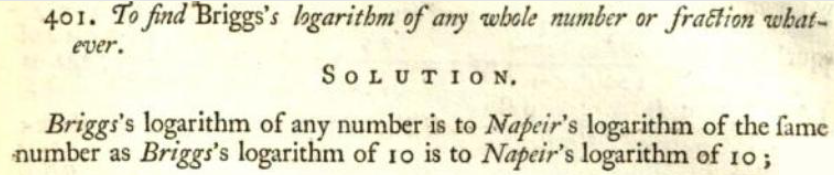The Briggs logarithm (also called the Briggsian logarithm, common logarithm, or decadic logarithm) is a logarithm to base 10; the natural logarithm (to base e) are called Naperian logarithms .

Note though, that the Briggs logarithm is denoted as Log(N) in the literature; the natural log is denoted as ln(N) .

As an example of a Brigg’s logarithm, the logarithm of 100 to the base 10 is 2. This is written as:

log10 100 = 2.

Note the “10” on the base: this tells you it is a Brigg’s logarithm.

## History of the Brigg’s LogarithmBriggs logarithm was mentioned in Saunderson and de Moivre’s 1740 text The Elements of Algebra .
Brigg’s logarithms are named after English mathematician Henry Briggs (1561–1630), who published, in 1624, the first table of common logarithms called Arithmetica Logarithmica; his table of logarithms went to 14 places of numbers from 1 to 20,000 and from 90,000 to 100,000 in 1624 .

Briggs logarithm tables allowed efficient replacement of multiplications by additions. One notable addition to the tables was the future Euler’s number (e), which was hidden in the tables in the limit e-1 = lim (1 – N-1)N, N → ∞ (Briggs never made its meaning explicit) .

The term “Briggs logarithm” was used in many early texts (1700s to 1800s). It fell out of common usage in the 1900s, replaced by the term “common logarithm.” However, you’ll still find the term used in mathematical texts, books on logarithms, and some specialized scientific fields.

Working with logarithms in general fell out of popular usage with the advent of powerful hand held calculators, which can handle every base. Instead of logarithms, calculators can solve exponential equations y = ax with the inverse x = log(to base a)y, which replaces the traditional “take the log of both sides.”

## References

 Logarithm. Retrieved March 6, 2022 from: http://www2.cfcc.edu/faculty/cmoore/LogarithmInfo.htm
 PROPERTIES OF THE POSITIVE INTEGERS.
Retrieved March 6, 2022 from: https://mae.ufl.edu/~uhk/ALL-ABOUT-INTEGERS.pdf
 Saunderson, N., Saunderson, J. & de Moivre, A. (1740). The Elements of Algebra, in Ten Books. University Press.
 Dani, S. Papadopoulis, A. (Eds.) (2019). Geometry in History. Springer.

CITE THIS AS:
Stephanie Glen. "Brigg’s Logarithm (Decadic logarithm)" From StatisticsHowTo.com: Elementary Statistics for the rest of us! https://www.statisticshowto.com/briggs-logarithm-decadic-logarithm/